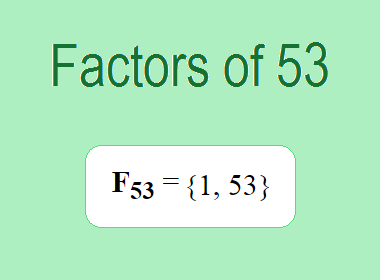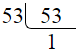# Factors of 53The factors of 53 are 1 and 53 i.e. F53 = {1, 53}. The factors of 53 are all the numbers that can divide 53 without leaving a remainder. 53 is a prime number, so it is divisible by 1 and 53 only.

We can check if these numbers are factors of 53 by dividing 53 by each of them. If the result is a whole number, then the number is a factor of 53. Let's do this for each of the numbers listed above:

·        1 is a factor of 53 because 53 divided by 1 is 53.

·        53 is a factor of 53 because 53 divided by 53 is 1.

## Properties of the Factors of 53

The factors of 53 have some interesting properties. One of the properties is that the sum of the factors of 53 is equal to 54. We can see this by adding all the factors of 53 together:

1 + 53 = 54

Another property of the factors of 53 is that the only prime factor of 53 is 53 itself.

********************

********************

## Applications of the Factors of 53

The factors of 53 have several applications in mathematics. One of the applications is in finding the highest common factor (HCF) of two or more numbers. The HCF is the largest factor that two or more numbers have in common. For example, to find the HCF of 53 and 106, we need to find the factors of both numbers and identify the largest factor they have in common. The factors of 53 are 1, and 53. The factors of 106 are 1, 2, 53, and 106. The largest factor that they have in common is 53. Therefore, the HCF of 53 and 106 is 53.

Another application of the factors of 53 is in prime factorization. Prime factorization is the process of expressing a number as the product of its prime factors. The prime factor of 53 is 53 since it is only the prime number that can divide 53 without leaving a remainder. Therefore, we can express 53 as:

53 = 53

We can do prime factorization by division method as given below,53 = 53

Since 53 is a prime number, there is no factor tree of 53.

## Conclusion

The factors of 53 are the numbers that can divide 53 without leaving a remainder. The factors of 53 are 1, and 53. The factors of 53 have some interesting properties, such as having a sum of 54. The factors of 53 have several applications in mathematics, such as finding the highest common factor and prime factorization.